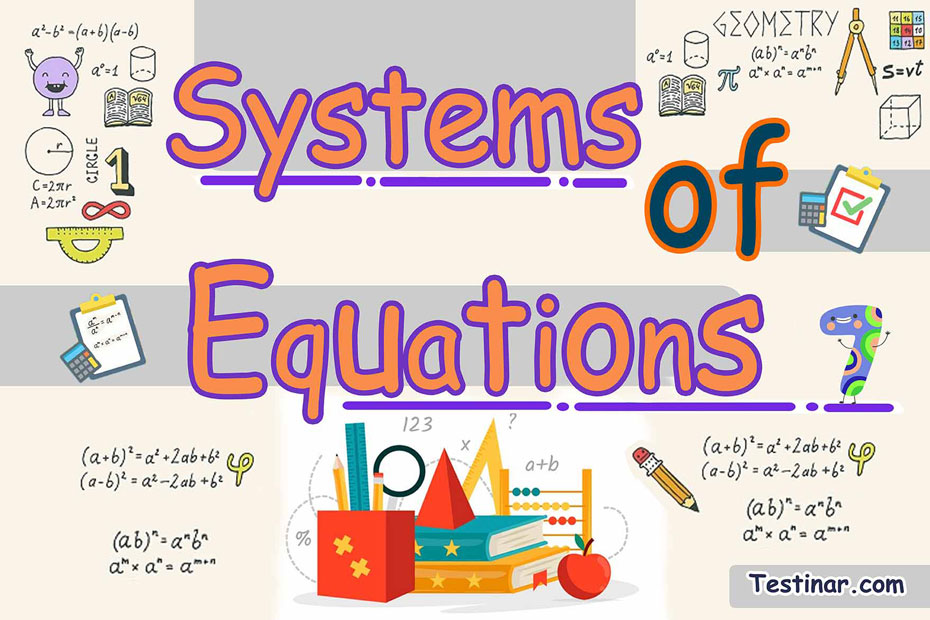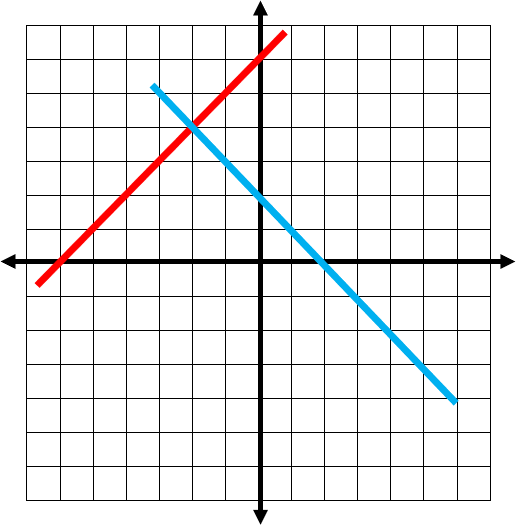## How to Solve Systems of Equations?

In maths, a system of equations additionally called a set of simultaneous or an equation system, is a fixed set of equations where we looked for common solutions. A system of equations may be categorized in a similar way as single equations. A system of equations can be applied in regular life by modeling problems in which any unknown values may be represented by variables.

### Definition of System of Equations

With algebra, a system of equations equals 2 or more equations and desires a common solution for these equations. "A system of linear equations equals a set of equations satisfied via the same set of variables."

### Example for a System of Equations

A system of equations like the one talked about previously which desires a common solution for the variables it includes. The below set of equations is one example of a system of equations,

• $$2x \ - \ y \ = \ 12$$
• $$x \ - \ 2y \ = \ 48$$

### Solving System of Equations Problems

Any kind of system of equations may be solved via varying techniques. In order to solve a system of equations in two variables, there must be two or more equations. Likewise, if trying to solve a system of equations in three variables, there must be three or more equations. So, let’s think about the three methods of solving a system of equations if the equations are linear equations in 2 variables. You would use these methods:

• Substitution
• Elimination
• Graphical

### Method 1: Solving a System of Equations by Substitution

To solve the system of equations via the substitution method if there are 2 linear equations in $$x$$ and $$y$$, show $$y$$ in terms $$x$$ in one of the equations, and after that substitute it in 2nd equation. Like this:

$$3x \ − \ y \ = \ 23 \ → \ (1)$$
$$4x \ + \ 3y \ = \ 48 \ → \ (2)$$
From($$1$$), we get: $$y \ = \ 3x \ − \ 23 \ → \ 3$$
Put in $$y$$ in ($$2$$), $$4x \ + \ 3(3x \ − \ 23) \ = \ 48 \ ⇒ \ 13x \ − \ 69 \ = \ 48 \ ⇒ \ 13x = 117 \ ⇒ \ x \ = \ 9$$
Now, put in $$x \ = \ 9$$ in ($$1$$): $$y \ = \ 3 \times 9 \ − \ 23 \ = \ 4$$
So, $$x \ = \ 9$$ and $$y \ = \ 4$$ is the answer to the provided system of equations.

### Method 2: Solving System of Equations by Elimination

Via the elimination method for solving a system of equations, you must get rid of one of the unknowns, via multiplying the equations by some kind of suitable numbers, therefore, the coefficients of one of the variables becomes the same as shown here:

$$2x \ + \ 3y \ = \ 4 \ → \ (1)$$ and $$3x \ + \ 2y \ = \ 11 \ → \ (2)$$
Coefficients of $$y$$ are $$3$$ and $$2$$; LCM ($$3$$, $$2$$) $$= \ 6$$
Multiplying Eqn($$1$$) by $$2$$ and Eqn($$2$$) by $$3$$, you end up with:
$$4x \ + \ 6y \ = \ 8 \ → \ (3)$$
$$9x \ + \ 6y \ = \ 33 \ → \ (4)$$
After subtracting ($$3$$) from ($$4$$), we get
$$5x \ = \ 25 \ ⇒ \ x \ = \ 5$$
Plut in $$x \ = \ 5$$ in ($$2$$) we get: $$15 \ + \ 2y \ = \ 11 \ ⇒ \ y \ = \ −2$$
so, $$x \ = \ 5$$, $$y \ = \ −2$$ is the answer.

### Method 3: Solving System of Equations by Graphical

With this technique, the answer for simultaneous equations is gotten via plotting their graphs. "The point of intersection of the two lines is the answer for the system of equations via the graphical method."

### Example

$$5x \ + \ 5y \ = \ 10$$
$$-1.5x \ + \ 1.5y \ = \ 9$$

Solution:

First, each equation should be graphed, to do that determine at least $$2$$ values of $$x$$ and $$y$$ which satisfy the equation $$5x \ + \ 5y \ = \ 10$$

 $$x$$ $$0$$ $$2$$ $$y$$ $$2$$ $$0$$

Likewise, determine at least $$2$$ values of $$x$$ and $$y$$ to satisfy the equation $$-1.5x \ + \ 1.5y \ = \ 9$$

 $$x$$ $$0$$ $$-6$$ $$y$$ $$6$$ $$0$$

By plotting those points on the graph you end up with the lines being in a coordinate plane as seen below.We see the two lines intersect at $$(-2 \ , \ 4)$$. Therefore, $$x \ = \ -2$$, $$y \ = \ 4$$ is the answer for the provided system of equations.

Methods 1 and 2 are an algebraic method of determining the answer for systems of equations and Method 3 is a graphical method.

### Exercises for Systems of Equations

1) \begin{align} 4x \ + \ 6y &= 22 \\ 7x \ + \ 6y &= 16 \\ \hline \end{align} $$\ \Rightarrow \$$

2) \begin{align} 2x \ + \ 5y &= 14 \\ 7x \ + \ 7y &= 28 \\ \hline \end{align} $$\ \Rightarrow \$$

3) \begin{align} 5x \ + \ 2y &= 13 \\ 4x \ + \ 6y &= 6 \\ \hline \end{align} $$\ \Rightarrow \$$

4)  \begin{align} 4x \ + \ 4y &= 0 \\ 3x \ + \ 7y &= -16 \\ \hline \end{align} $$\ \Rightarrow \$$

5) \begin{align} 5x \ + \ 6y &= 44 \\ 6x \ + \ 7y &= 52 \\ \hline \end{align} $$\ \Rightarrow \$$

6) \begin{align} 2x \ + \ 4y &= 14 \\ 7x \ + \ 4y &= 39 \\ \hline \end{align} $$\ \Rightarrow \$$

7)  \begin{align} 4x \ + \ 2y &= -24 \\ 5x \ + \ 2y &= -28 \\ \hline \end{align} $$\ \Rightarrow \$$

8)  \begin{align} 4x \ + \ 7y &= 5 \\ 7x \ + \ 3y &= -19 \\ \hline \end{align} $$\ \Rightarrow \$$

9) \begin{align} 4x \ + \ 7y &= -22 \\ 6x \ + \ 4y &= -20 \\ \hline \end{align} $$\ \Rightarrow \$$

10) \begin{align} 3x \ + \ 3y &= 0 \\ 3x \ + \ 6y &= 0 \\ \hline \end{align} $$\ \Rightarrow \$$

1) \begin{align} 4x \ + \ 6y &= 22 \\ 7x \ + \ 6y &= 16 \\ \hline \end{align} \ \Rightarrow \color{red}{ + \begin{align} -7(4x \ + \ 6y) &= -7(22) \\ 4(7x \ + \ 6y) &= 4(16) \\ \hline -18y &= -90 \end{align}} $$\ \Rightarrow \ \color{red}{ y = \frac{-90 }{ -18} = 5}$$$$\ \Rightarrow \ \color{red}{ 4x \ + \ 6(5) = 22 }$$ $$\ \Rightarrow \ \color{red}{ x = \frac{22 \ - \ (30)}{4} = -2}$$
2) \begin{align} 2x \ + \ 5y &= 14 \\ 7x \ + \ 7y &= 28 \\ \hline \end{align} \ \Rightarrow \color{red}{ + \begin{align} -7(2x \ + \ 5y) &= -7(14) \\ 2(7x \ + \ 7y) &= 2(28) \\ \hline -21y &= -42 \end{align}} $$\ \Rightarrow \ \color{red}{ y = \frac{-42 }{ -21} = 2}$$$$\ \Rightarrow \ \color{red}{ 2x \ + \ 5(2) = 14 }$$ $$\ \Rightarrow \ \color{red}{ x = \frac{14 \ - \ (10)}{2} = 2}$$
3) \begin{align} 5x \ + \ 2y &= 13 \\ 4x \ + \ 6y &= 6 \\ \hline \end{align} \ \Rightarrow \color{red}{ + \begin{align} -4(5x \ + \ 2y) &= -4(13) \\ 5(4x \ + \ 6y) &= 5(6) \\ \hline 22y &= -22 \end{align}} $$\ \Rightarrow \ \color{red}{ y = \frac{-22 }{ 22} = -1}$$$$\ \Rightarrow \ \color{red}{ 5x \ + \ 2(-1) = 13 }$$ $$\ \Rightarrow \ \color{red}{ x = \frac{13 \ - \ (-2)}{5} = 3}$$
4) \begin{align} 4x \ + \ 4y &= 0 \\ 3x \ + \ 7y &= -16 \\ \hline \end{align} \ \Rightarrow \color{red}{ + \begin{align} -3(4x \ + \ 4y) &= -3(0) \\ 4(3x \ + \ 7y) &= 4(-16) \\ \hline 16y &= -64 \end{align}} $$\ \Rightarrow \ \color{red}{ y = \frac{-64 }{ 16} = -4}$$$$\ \Rightarrow \ \color{red}{ 4x \ + \ 4(-4) = 0 }$$ $$\ \Rightarrow \ \color{red}{ x = \frac{0 \ - \ (-16)}{4} = 4}$$
5) \begin{align} 5x \ + \ 6y &= 44 \\ 6x \ + \ 7y &= 52 \\ \hline \end{align} \ \Rightarrow \color{red}{ + \begin{align} -6(5x \ + \ 6y) &= -6(44) \\ 5(6x \ + \ 7y) &= 5(52) \\ \hline -1y &= -4 \end{align}} $$\ \Rightarrow \ \color{red}{ y = \frac{-4 }{ -1} = 4}$$$$\ \Rightarrow \ \color{red}{ 5x \ + \ 6(4) = 44 }$$ $$\ \Rightarrow \ \color{red}{ x = \frac{44 \ - \ (24)}{5} = 4}$$
6) \begin{align} 2x \ + \ 4y &= 14 \\ 7x \ + \ 4y &= 39 \\ \hline \end{align} \ \Rightarrow \color{red}{ + \begin{align} -7(2x \ + \ 4y) &= -7(14) \\ 2(7x \ + \ 4y) &= 2(39) \\ \hline -20y &= -20 \end{align}} $$\ \Rightarrow \ \color{red}{ y = \frac{-20 }{ -20} = 1}$$$$\ \Rightarrow \ \color{red}{ 2x \ + \ 4(1) = 14 }$$ $$\ \Rightarrow \ \color{red}{ x = \frac{14 \ - \ (4)}{2} = 5}$$
7) \begin{align} 4x \ + \ 2y &= -24 \\ 5x \ + \ 2y &= -28 \\ \hline \end{align} \ \Rightarrow \color{red}{ + \begin{align} -5(4x \ + \ 2y) &= -5(-24) \\ 4(5x \ + \ 2y) &= 4(-28) \\ \hline -2y &= 8 \end{align}} $$\ \Rightarrow \ \color{red}{ y = \frac{8 }{ -2} = -4}$$$$\ \Rightarrow \ \color{red}{ 4x \ + \ 2(-4) = -24 }$$ $$\ \Rightarrow \ \color{red}{ x = \frac{-24 \ - \ (-8)}{4} = -4}$$
8) \begin{align} 4x \ + \ 7y &= 5 \\ 7x \ + \ 3y &= -19 \\ \hline \end{align} \ \Rightarrow \color{red}{ + \begin{align} -7(4x \ + \ 7y) &= -7(5) \\ 4(7x \ + \ 3y) &= 4(-19) \\ \hline -37y &= -111 \end{align}} $$\ \Rightarrow \ \color{red}{ y = \frac{-111 }{ -37} = 3}$$$$\ \Rightarrow \ \color{red}{ 4x \ + \ 7(3) = 5 }$$ $$\ \Rightarrow \ \color{red}{ x = \frac{5 \ - \ (21)}{4} = -4}$$
9) \begin{align} 4x \ + \ 7y &= -22 \\ 6x \ + \ 4y &= -20 \\ \hline \end{align} \ \Rightarrow \color{red}{ + \begin{align} -6(4x \ + \ 7y) &= -6(-22) \\ 4(6x \ + \ 4y) &= 4(-20) \\ \hline -26y &= 52 \end{align}} $$\ \Rightarrow \ \color{red}{ y = \frac{52 }{ -26} = -2}$$$$\ \Rightarrow \ \color{red}{ 4x \ + \ 7(-2) = -22 }$$ $$\ \Rightarrow \ \color{red}{ x = \frac{-22 \ - \ (-14)}{4} = -2}$$
10) \begin{align} 3x \ + \ 3y &= 0 \\ 3x \ + \ 6y &= 0 \\ \hline \end{align} \ \Rightarrow \color{red}{ + \begin{align} -3(3x \ + \ 3y) &= -3(0) \\ 3(3x \ + \ 6y) &= 3(0) \\ \hline 9y &= 0 \end{align}} $$\ \Rightarrow \ \color{red}{ y = \frac{0 }{ 9} = 0}$$$$\ \Rightarrow \ \color{red}{ 3x \ + \ 3(0) = 0 }$$ $$\ \Rightarrow \ \color{red}{ x = \frac{0 \ - \ (0)}{3} = 0}$$

## Systems of Equations Practice Quiz

### TSI Mathematics Formulas

$6.99$5.99

### CLEP College Math in 10 Days

$24.99$13.99

### ATI Teas Math in 7 Days

$18.99$14.99

### 5 Full Length SSAT Lower Level Math Practice Tests

$16.99$11.99